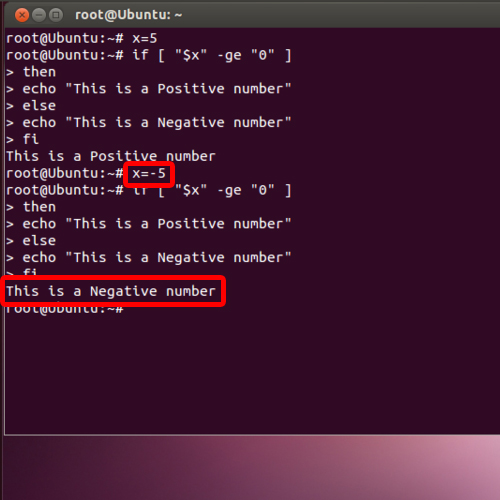## How to Use Conditions in Bash Scripting (Bash IF Script)

In this tutorial we will show you how to write a bash if script. This allows us to add conditions to the commands we enter. Through this we can also command bash to give an alternate output.
Scripting in bash can become fairly easy once you get the hang of it. To learn how to write a Bash IF command and script follow the steps given below.

Step 1 – Give the variable a positive value

First of all, we will define the value of the variable over here. For now, let’s give it a positive value.
x=5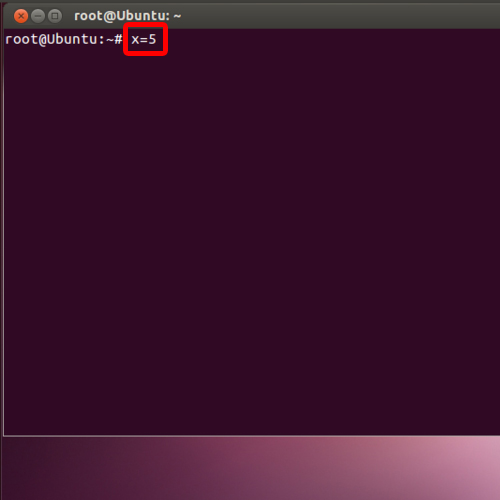Step 2 – Put condition on the variable

With that done, let’s start with the “if” function. Now we will put a condition on the variable which we had defined earlier, that if the input value is greater than or equal to zero, then we will print the following message on the screen.
If [ “\$x” –ge “0” ]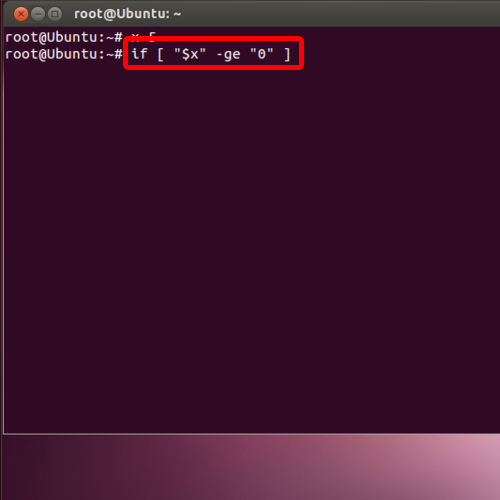Step 3 – Add keyword to display alternate message

After that, we will use the else keyword, to display the alternate message if the condition is false. Over here, we will display the following message if the condition is not true.
then
echo “This is a Positive number”
else
echo “This is a Negative number”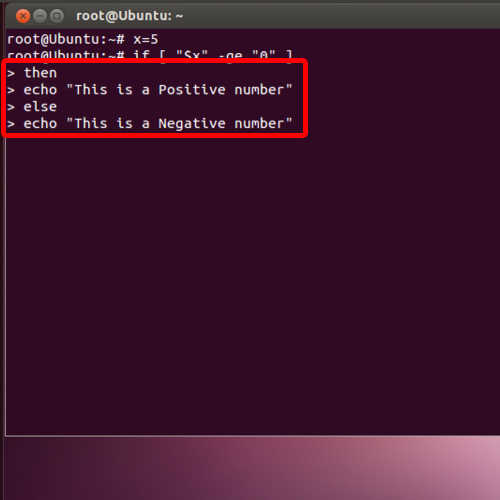Step 4 – Run the script

Now let’s close the condition and run the bash if script that we just created. Great! The script runs perfectly.

fi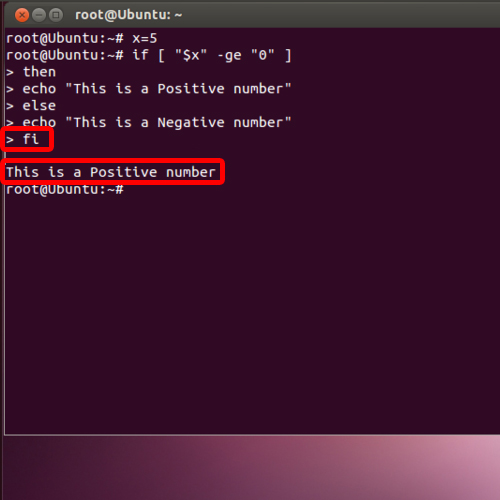Step 5 – Input “-5”

Similarly, if we input “-5”, you can see that the alternate message is displayed on the screen.
x = -5
From this you can see that scripting in bash is not complicated if you know the correct usage of your commands.